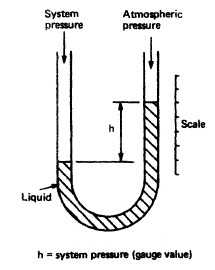# Day 87

Pressure is defined as force per unit area.

P = F/A

Pressure is created when the particles of a substance continually push against the walls of its container or objects immersed in it. The pressure of fluids varies with depth. For an object immersed in a fluid, the average pressure in the fluid at the level to which the object has been submerged is defined as the ratio of force to area (P = F/A).

We live in a fluid (the atmosphere). The farther up you are in our atmosphere, the less the air pressure. However, all points at the same depth in a fluid must be at the same pressure. So all points at sea level on Earth are at the same pressure (atmospheric pressure at sea level = 1.013 X 105 Pa or 14.7 psi).

In SI, pressure is measured in Pascals (Pa). One Pa = 1 N/m2. In English, pressure is measured in pounds per square inch (psi).

Pressure not only varies with depth, it also varies with the area in contact. If you stand on a platform, you are exerting a certain force (your weight) over a relatively small area (the area of the soles of your feet).

However, is you lie down on the platform, although your force (weight) remains the same, there is contact over a much larger area so the pressure is decreased.

Consider an open container of fluid. The pressure at the top of the fluid is atmospheric pressure at that level.

As you go down into the container, you add the pressure of the fluid to air pressure. The pressure at a depth h below the surface of a liquid open to the atmosphere is greater than the atmospheric pressure by the amountgh.

(= density).

Any increase in pressure at the surface of an enclosed fluid must be transmitted to every point in the fluid. This is known as Pascals Principle and is the underlying premise behind hydraulics.

A simple manometer (open tube device) is used to measure pressure.SourceP = Po + pgh

In medical applications, a sphygmomanometer (a specialized manometer) is used to measure blood pressure.

Note:   Gauge pressure P0 = pressure difference between fluid pressure and atmospheric pressure

Actual pressure, P = gauge pressure plus the atmospheric pressure (Patm)

Therefore: P = P0 + ρgh (Pressure – depth relationship equation found on your equation sheet)

Standard Patm = 1.0 x 105 Pa = 1 atm = 14.7 psi = 760 Torr = 760 mm Hg

(source)